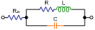# Calculate Magnitude of Impedance for RLC Circuit

• Goerge

#### Goerge

I need help calculating the magnitude of the impedance for a RLC circuit shown below. See the attached schematic.

I found the impedance magnitude of a similar circuit here:
Impedance Formulas

...see the 4th circuit from the bottom (let's call its impedance Z_a).

Unfortunately, I do not know how to include the series resistance Rx into this formula.
The problem I am having is whether to use the formula:
Z = Rx + Z_a
...or
Z = (Rx^2 + ?)^0.5

Also, I am not sure whether the value of the Rx will alter the phase angle between current and voltage of this circuit?

#### Attachments

•ZZZ.png
778 bytes · Views: 446
First lump R and L into an equivalent series impedance, call it Z1, that will be a complex number: Z1 = R+jwL

So now you have an impedance Z1 in parallel with C.

Next, lump the equivalent series impedance found above with the capacitor in parallel into a new equivalent impedance Z2, remembering that the complex impedance of a capacitor is 1/(jwC): Z2 = (Z1 * (1/(jwC)))/(Z1 + 1/(jwc))

The last lumping is to combine Rx and Z2 as series impedances: Rx + Z2. It will help to get Z2 in terms of a real and complex part (manipulate the thing algebraically) so that you can just add Rx to the real part of Z2.

Now you can simplify this complex impedance to get a real and a complex term. Now you have the real and imaginary parts of the total circuit, and you use the magnitude formula sqrt(real^2 + img^2) to get the magnitude.